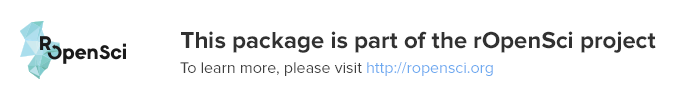# colocr

An R package for conducting co-localization analysis.

## Overview

A few R packages are available for conducting image analysis, which is a very wide topic. As a result, some of us might feel at a loss when all they want to do is a simple co-localization calculations on a small number of microscopy images. This package provides a simple straight forward workflow for loading images, choosing regions of interest (ROIs) and calculating co-localization statistics. Included in the package, is a shiny app that can be invoked locally to interactively select the regions of interest in a semi-automatic way. The package is based on the R package `imager`.

## Installing `colocr`

`colocr` is available on CRAN and can be installed using

``````# install from cran
install.packages('colocr')``````

The package development version is available at github.

``````# install from github
devtools::install_github('ropensci/colocr')``````

This package depends on `imager` which has some external dependencies. The instructions for installing `imager` can be found here.

## Getting started

To get started, load the required packages and the images. The images below are from DU145 cell line and were stained for two proteins; RKIP and LC3. Then, apply the appropriate parameters for choosing the regions of interest using the `roi_select`. Finally, check the appropriateness of the parameters by highlighting the ROIs on the image.

``````# load libraries
library(colocr)

fl <- system.file('extdata', 'Image0001_.jpg', package = 'colocr')

# select ROI and show the results
par(mfrow = c(2,2), mar = rep(1, 4))

img %>%
roi_select(threshold = 90) %>%
roi_show()``````

The same can be achieved interactively using an accompanying shiny app. To launch the app run.

``run_app()``

The reset of the analysis depends on the particular kind of images. Now, `colocr` implements two simple co-localization statistics; Pearson’s Coefficient Correlation (PCC) and the Manders Overlap Coefficient (MOC).

To apply both measures of correlation, we first get the pixel intensities and call `roi_test` on the merge image.

``````# calculate co-localization statistics
img %>%
roi_select(threshold = 90) %>%
roi_test(type = 'both')``````

The same analysis and more can be conducted using a web interface for the package available here

## More

``browseVignettes('colocr')``